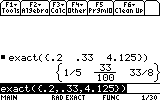# Knowledge Base

## Solution 13342: Using the Exact() Function on the TI-89 Family, TI-92 Family and Voyage™ 200 Graphing Calculators.

### How do I use the exact() function on my TI-89 family, TI-92 family or Voyage 200 graphing calculator?

The proper syntax for the exact() function are listed below.

exact(expression1[,tol])
exact(list1[,tol])
exact(matrix1[,tol])

The exact() function ensures that the calculator uses the Exact mode arithmetic regardless of the Exact/Approx mode setting to return, when possible, the rational-number equivalent of the argument.

Note: tol specifies the tolerance for the conversion; the default is set to 0(zero).

Refer to the examples provided below.

Ex.1 exact(.25) [ENTER]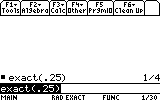Ex.2 exact(.333333) [ENTER]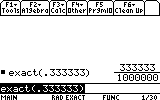Ex.3 exact(.33333, .001) [ENTER]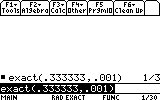Ex.4 exact(3.5x+y) [ENTER]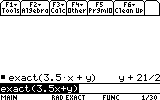Ex.5 exact({.2, .33, 4.125}) [ENTER]